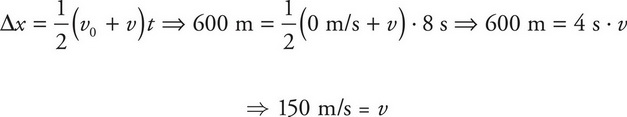# AP Physics 1 Question 380: Answer and Explanation

### Test Information

Question: 380

8. A car initially at rest accelerates linearly at a constant rate for eight seconds. If the total displacement of the car was 600 m, what was the speed of the car after the eight seconds of acceleration?

• A. 50 m/s
• B. 100 m/s
• C. 150 m/s
• D. 200 m/s